# La Peste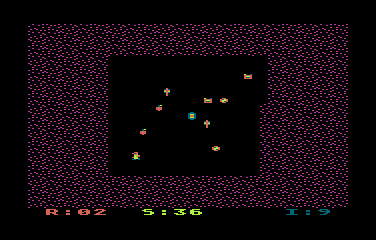This is a little game for the 2018 NOMAM's BASIC 10-liners Contest. This program fits in the EXTREM-256 category, and it was written using FastBasic 3.4 for the 8-bits ATARI XL/XE. Development started on 2018-02-17, and it took 6 days. The final version's date is 2018-02-27.

UPDATE: It obtained the 4th place of 17 entries in the category.

## Description

Save as many items as you can in your bunker before they become infected with the purple plague.

## Instructions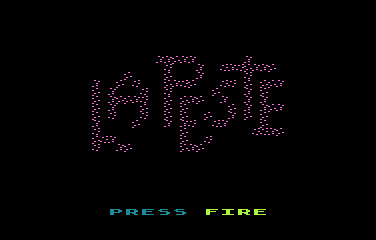When you run the game, you'll get the intro screen. Press the trigger in joystick 1 to start.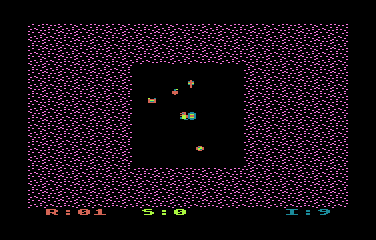You are the small guy besides the door of a bunker, and you have to collect the items before the purple plague catch them.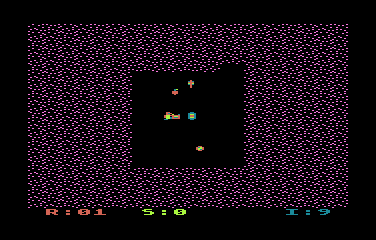The items must be pushed into the bunker.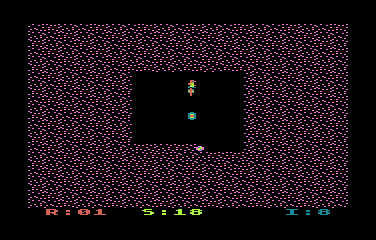When an item is reached by the plague, the global spare items number decreases. The game will finisg if there are no more spare items.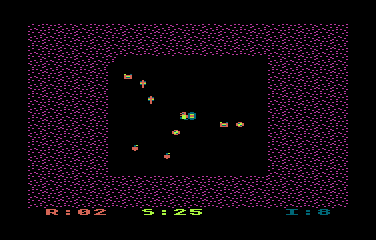When there are no more unsafe items in the screen, the next region, a bigger one with more objects, must be also cleared.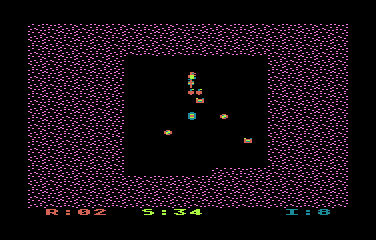It's a good idea to move the items that are closer to the plague before pushing them into the bunker, but be carefull: don't place too many items together, or you wont be able to push them later.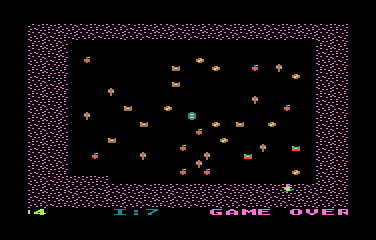If the plague reaches you or you fall into the plague, you'll dead and the game is over.

Get the LAPESTE.ATR file and set it as drive 1 in a real Atari (or emulator). Turn on the computer and the game should start after loading.

## The code

The abbreviated BASIC code is the following. Please note that FastBasic does not use line numbers!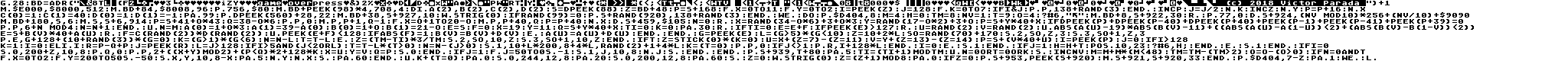The full and expanded BASIC listing is:

 ```graphics 28 ``` Sets graphics mode 12 without text box. This is ANTIC mode 4 with 40x24 chars of 4x8 4-color pixels. ```bd=adr("{data}")+1 ``` Binary data: 1-4 (4 bytes): NTSC palette 5-8 (4 bytes): PAL palette 9-26 (18 bytes): score line 27-29 (3 bytes): filler 30-38 (9 bytes): "game over" message 39-48 (10 bytes): "start" message 49-84 (36 bytes): intro logo 85-180 (96 bytes): charset 181-186 (6 bytes): plague pattern to fill the screen 187-210 (24 bytes): copyright ;-) ```move \$E000,\$8000,512 move bd+84,\$8008,96 poke 756,\$80 ``` Sets up the new charset in RAM. The modified internal codes are: 0: Ground. 1: Bunker. 2-5: Player in 4 different shapes. 6-9: 4 items. 10-12: 3 different plague patterns. 128+: Any of the previous chars changes the color when it is in "video inverted" mode to show them as infected. ```move bd+peek(98)*4,708,4 ``` Sets the colors using the proper palette. ```dim a(2),b(2),c(2),d(2) ``` Defines the arrays. ```s=dpeek(88) ``` Sets the base address of screen data. ```z=bd+48 p=s+168 for x=0 to 11 for y=0 to 2 i=peek(z) j=128 for k=0 to 7 if i&j poke p,138+rand(3) endif inc p j=j/2 next k inc z next y p=p+16 next x ``` Decodes the title screen. It places a randomly selected plague char for every "true" bit in data. ```c(0)=1 c(1)=40 d(0)=1 d(1)=-1 ``` Sets the constants for the plague algorithm. ```pause 99 ``` Just a pause before the title animation. ```poke dpeek(560)+28,22 ``` Changes the botom line from ANTIC mode 4 to ANTIC mode 6 with the horizontal fine scroll bit enabled. ```move bd+38,s+927,10 ``` Displays the "press fire" message in the title screen. ```while strig(0) if rand(99)=0 poke s+rand(920),138+rand(3) endif wend ``` Waits for the trigger while adding spots of plague over the title screen. ```do ``` Main loop. ``` poke \$D404,8 ``` Sets the horizontal fine scroll to the desired shift. ``` m=4 h=0 tm=8 nv=1 t=9 o=4 ``` Initializes game variables: M: Max number of items from a region. H: Score. TM: Timer for every propagation. The lower, the faster... NV: Number of the region (round). T: Number of the spare items. Decreases each time an item gets infected. O: Size of the safe area of a region. ``` ? #6,"{chr 125}" move bd+8,s+922,30 ``` Cleans the screen and puts the score line at the bottom of it. ``` repeat ``` Game loop. ``` poke 77,0 ``` Resets/disables the ATRACT mode. ``` dpoke s+924,(nv mod 10)*256+(nv/10)+\$9090 ``` Prints the current region number (round). ``` move bd+180,s,6 move s,s+6,914 ``` Fills the screen with the plague pattern<./td> ``` p=s+41+o*43 q=38-o*6 poke p,0 move p,p+1,q-1 for x=o+1 to 20-o move p,p+40,q p=p+40 next x ``` Cleans the center of the playfiels. The initial size of the safe areas is based on the round number. ``` dpoke s+459,\$105 ``` Puts the player and the bunker in the middle of the playfield. ``` n=0 repeat x=rand(34-o*6)+3+o*3 y=rand(17-o*2)+3+o p=s+y*40+x if dpeek(p)+dpeek(p-40)+dpeek(p+40)+ peek(p-1)+peek(p-41)+peek(p+39)=0 poke p,6+n mod 4 n=n+1 endif until n=m ``` Places the items randomly in the playfield, leaving an initial double path between the items and the plague, but it also checks that there is always space around them. ``` a(0)=1+o*3 a(1)=39-a(0) b(0)=1+o b(1)=21-o ``` Sets the initial coordinates of the safe zone in the playfield. A()=horizontal, B()=vertical, 0=upper-left, 1=lower-right. ``` e=s f=0 ``` Sets initial position of the plague propagation over the upper-left corner of the playfield, and the direction as the same place. This forces a new propagation wave at the round start. ``` x=19 y=11 q=s+y*40+x ``` Sets the initial coordinates of the player. ``` ti=0 ``` Sets the initial value of the timer counter. ``` k=0 ``` K is the "killed" flag. Set to 1 when the game should finish by some event. ``` pause 30 ``` A small pause before the begining of the round. ``` repeat ``` Round loop. ``` if ti=0 ``` Is it time to propagate? ``` e=e+f ``` Calculates the next step of the propagation. ``` if peek(e)>127 ``` If it is already infected... ``` repeat u=rand(2) v=rand(2) until abs(a(u)-19)*abs(b(v)-11) + ((abs(a(u)-a(1-u))<2)+(abs(b(v)-b(1-v))<2)) e=s+b(v)*40+a(u) ``` Selects a corner to start a new propagation wave. It can't be a row or column where the bunker is. ``` repeat f=c(rand(2))*d(rand(2)) until peek(e+f)<128 ``` Selects a propagation direction. It can't be towards an already infected area. ``` if abs(f)=1 b(v)=b(v)+d(v) else a(u)=a(u)+d(u) endif ``` Adjusts the limits of the safe area. ``` endif ``` ``` g=peek(e) l=(g>5)*(g<10) ``` Checks if there is an item in the newly infected location. ``` z=10+2*l so=rand(70)+170 sound 2,so,z,3 sound 3,so+1,z,3 ``` Plays a tone each propagation step. There is not a pure tone if an item was infected. ``` poke e,g+128+(10+rand(3))*(g=0) ``` If there is an item, it changes the main color to purple by adding 128 to its ATASCII code. If it is an empty space, one of the different patterns of the plague. ``` k=(g>1)*(g<6) ``` Checks if the plague killed the player (in any of the 4 different sprites). ``` n=n-l t=t-l ``` If an item was infected, decreases the number of items that have to be collected and the global number of remaining spare items. ``` else ``` If it is not time to propagate... ``` z=(tm-ti)*3/tm sound 2,so,10,z sound 3,so+1,10,z endif ``` , just lowers the volume of the tone. ``` if t ``` If there are spare items... ``` z=stick(0)*(k=0) u=x+(z=7)-(z=11) v=y+(z=13)-(z=14) p=s+(v*40+u) ``` If the player is still alive, gets the new coordinates of the player and the location in the screen. ``` i=peek(p) ``` Gets what is in that location. ``` j=0 ``` ``` if i>128 k=1 i=0 ``` If there is an infected item or the plague, sets the "killed" flag and allows the movement of the player to that new location. ``` elif i r=p-q+p j=peek(r) ``` If there is a non-infected item in the new location, checks what is behind that item. ``` l=j>128 ``` Checks if the item behind the item near the player is infected. ``` if i>5 and (j<2 or l) ``` If it is posible to push an item: ``` t=t-l*(t>0) ``` decreases the number of total spare items if it was pushed over an infected item or the plague, ``` n=n-(j>0) ``` and decreases the number of remaining items unless it was pushed into an empty space. ``` sound 1,10+l*200,8+4*l,rand(2)+1+4*l ``` Plays a sound based on the movement of the player and its effect. It might be rubbing the item with the floor or pushing into infected area. ``` k=(t=0) ``` Sets the "killed" flag if there are no more spare items. ``` poke p,0 ``` Cleans the new position of the player. ``` if j<>1 poke r,i+128*l endif ``` If the bunker is not behind the item, puts it there, but in the infected mode if there was an infected item or the plague behind it. ``` i=0 ``` Enables the space to move the player. ``` else sound 1 endif ``` If it was not possible to push an item, turns off the sound. ``` if j=1 h=h+t position 10,23 ?#6,h; endif ``` If the bunker is behind the pushed item, increases the score by the number of remaining spare items. ``` else sound 1 endif ``` Turns off the rubbing sound. ``` if i=0 sound 0,200+z,10,8 poke q,0 poke p,2+((x+y) mod 2)+(p2) o=o-(o>0) ``` Increments the round number, doubles the number of items, reduces the timer and enlarges the size of the safe area in the playfield. ``` if n=0 and t for x=0 to 2 for y=200 to 50 step -50 sound x,y,10,8-x pause 5 next y next x sound pause 60 endif ``` Just plays a tune when a round was completed and it is possible to continue with the next round. ``` until k+(t=0) ``` The game ends when the player was infected or when there are no more spare items. ``` pause 0 sound 0,244,12,8 pause 20 sound 0,200,12,8 pause 60 sound ``` Plays an end-of-game tune, which are just two tones. ``` z=0 while strig(0) z=(z+1) mod 8 pause 0 if z=0 poke s+953,peek(s+920) move s+921,s+920,33 endif poke \$D404,7-z pause 1 wend ``` Waits for the trigger to start a new game while the bottom line is fine-scrolled to show the "game over" message and . ```loop ``` End of game loop... restart!